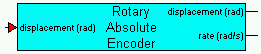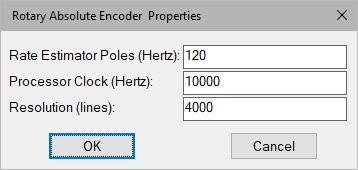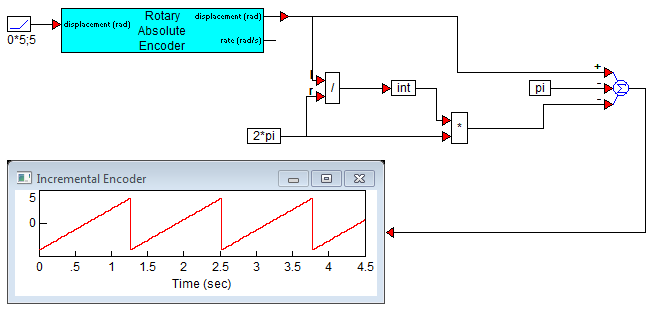### Rotary Absolute EncoderCategory:  Sensors

The Rotary Absolute Encoder block models quantized displacement measurements obtained from a generic encoding device such as a rotary optical encoder or similar device. This block provides simulation of processor rate estimation from quantized signal.Rate Estimator Poles: Defines the bandwidth of rate estimation. This option typically requires a trade-off between rate estimation accuracy and noise. Units are in hertz.

Processor Clock: Defines the processor clock rate for rate estimation.

Resolution: Indicates the total number of lines per revolution.

Displacement (input): Indicates the physical angular displacement connection. Units are in rad.

Displacement (output): Indicates from where the quantized displacement measurement is read. Units are in rad.

Rate: Indicates from where the estimated rate is read. Units are in rad/sec.

#### Example

Diagram name: Rotary Absolute Encoder

Location: Examples > eMotor > Sensor

The Rotary Encoder block simulates an incremental encoder with an assumed built-in counter providing undisturbed unlimited displacement output. A true absolute encoder that uses some type of binary coded scales will reset to 0 position after one complete revolution, and will usually issue an index signal at the 0 position to signal an external counter or processor. In cases where a simulation of an absolute encoder is needed, a simple block construction can be made to calculate absolute angular position using a modulo 2π conversion. The following simulation example illustrates this method. In this example, π is subtracted at the output to provide ±π measurements.